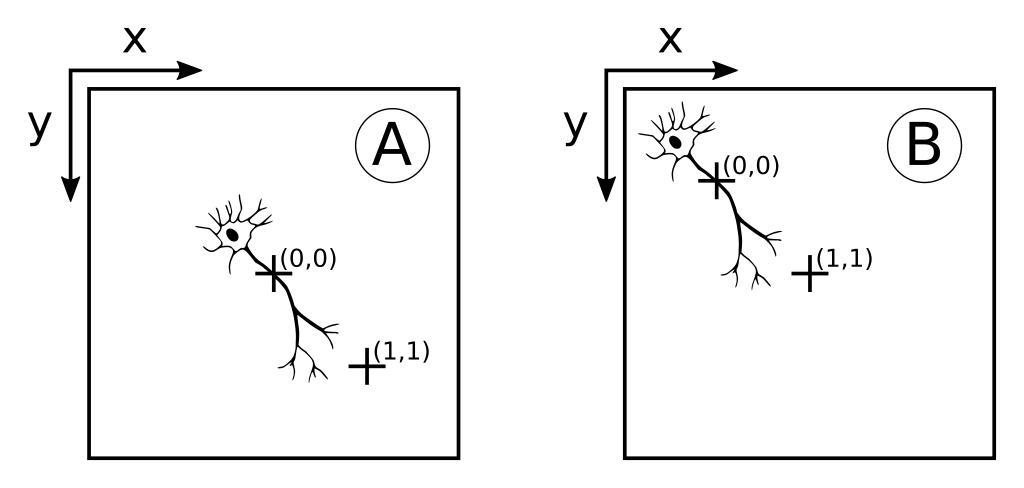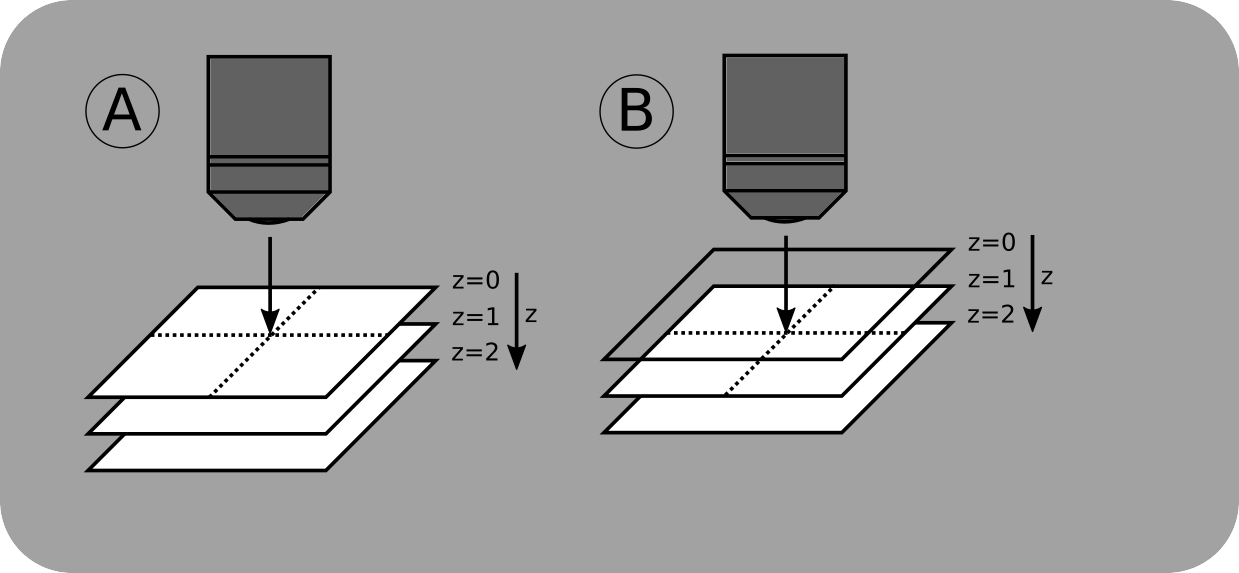# Sample Coordinate System¶

The ScanImage sample coordinate system defines a coordinate system within the sample under investigation. The ScanImage sample coordinate system is typically aligned with the X/Y/Z axes of the microscope stage. The units of the sample coordinate system is microns. The origin of the sample coordinate system can be freely chosen by clicking the ‘Zero’ buttons in the Motor Controls. It is defined as a right handed coordinate system where the positive z axis points into the sample (away from the objective). It is important to configure ScanImage’s motor system to follow this convention (see images on right).

## Motor Controls¶The Motor Controls window shows the current Z-Focusdepth in Sample Coordinates at 150um. The focus depth is a combination of the sample position (100um) and the position of the piezo objective positioner (50um). The Stack Controls window shows the slice positions in sample coordinates.

## Sample Coordinates: Lateral¶ScanImage® images the sample at lateral coordinates (0,0) ScanImage® images the sample at lateral coordinates (1,1)

When incrementing the X and Y sample coordinates, the sample will move toward the top left corner of the viewport.

## Sample Coordinates: Axial¶ScanImage® images the sample at axial coordinates z = 0 ScanImage® images the sample at axial coordinates z = 1

By incrementing the Z sample coordinate, the focal point moves into the sample.

## API¶

Use the following commands to interact with the stage or coordinate system.

```xyz = [0 0 0];
hSI.hMotors.moveSample(xyz); % move the focal point to the sample position specified x,y,z coordinates
```
```hSI.hMotors.queryPosition(); % query the motor positions
```
```position = hSI.hMotors.samplePosition; % read the current focal position in the sample coordinate space
```
```hSI.hMotors.zeroSample(); % establish relative origin
```
```hSI.hMotors.clearZero(); % clear relative origin
```